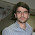Support us .Net Basics C# SQL ASP.NET ADO.NET MVC Slides C# Programs Subscribe Buy DVD

### Part 5 - Aggregate function in LINQ

Suggested Videos
Part 2 - Writing LINQ Queries
Part 3 - Extension Methods
Part 4 - LINQ Aggregate Functions

In this video we will discuss the use of Aggregate() LINQ function. In Part 4 of LINQ Tutorial, we discussed the following functions.
Min
Max
Sum
Count
Average

Let us understand the use of Aggregate() function with examples.

Example 1: Consider the following string array.
string[] countries = { "India", "US", "UK", "Canada", "Australia" };

We want to combine all these strings into a single comma separated string. The output of the program should be as shown below.
India, US, UK, Canada, Australia

Without LINQ, the program will be as shown below.
using System;
namespace Demo
{
class Program
{
static void Main()
{
string[] countries = { "India", "US", "UK", "Canada", "Australia" };

string result = string.Empty;
for (int i = 0; i < countries.Length; i++)
{
result = result + countries[i] + ", ";
}

int lastIndex = result.LastIndexOf(",");
result = result.Remove(lastIndex);

Console.WriteLine(result);
}
}
}

With LINQ Aggregate function
using System;
using System.Linq;
namespace Demo
{
class Program
{
static void Main()
{
string[] countries = { "India", "US", "UK", "Canada", "Australia" };

string result = countries.Aggregate((a, b) => a + ", " + b);

Console.WriteLine(result);
}
}
}

How Aggregate() function works?
Step 1. First "India" is concatenated with "US" to produce result "India, US"
Step 2. Result in Step 1 is then concatenated with "UK" to produce result "India, US, UK"
Step 3: Result in Step 2 is then concatenated with "Canada" to produce result "India, US, UK, Canada"

This goes on until the last element in the array to produce the final single string "India, US, UK, Canada, Australia"

Example 2: Consider the following integer array
int[] Numbers = { 2, 3, 4, 5 };

Compute the product of all numbers

Without LINQ
using System;
namespace Demo
{
class Program
{
static void Main()
{
int[] Numbers = { 2, 3, 4, 5 };

int result = 1;
foreach (int i in Numbers)
{
result = result * i;
}

Console.WriteLine(result);
}
}
}

With LINQ:
using System;
using System.Linq;
namespace Demo
{
class Program
{
static void Main()
{
int[] Numbers = { 2, 3, 4, 5 };

int result = Numbers.Aggregate((a, b) => a * b);

Console.WriteLine(result);
}
}
}

How Aggregate() function works?
Step 1: Multiply (2X3) to produce result 6
Step 2: Result (6) in Step 1 is then multiplied with 4 (6X4) to produce result 24
Step 3: Result (24) in Step 2 is then multiplied with 5 (24X5) to produce final result 120

Example 3: Consider the following integer array
int[] Numbers = { 2, 3, 4, 5 };

One of the overloaded version of Aggregate() function has a Seed parameter. If we pass 10 as the value for Seed parameter
int result = Numbers.Aggregate(10, (a, b) => a * b);

1200 will be the result

Step 1: Multiply (10X2) to produce result 20
Step 2: Result (20) in Step 1 is then multiplied with 3 (20X3) to produce result 60
Step 3: Result (60) in Step 2 is then multiplied with 4 (60X4) to produce result 240
Step 4: Result (240) in Step 3 is then multiplied with 5 (240X5) to produce final result 12001.2.